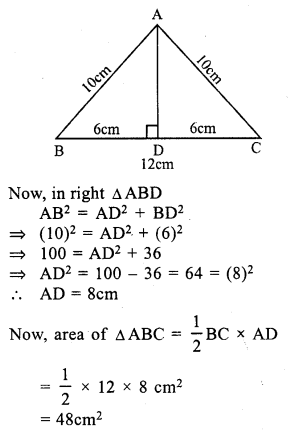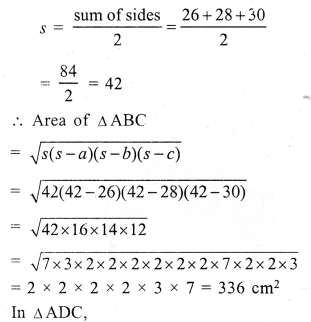# RS Aggarwal Class 7 Solutions Chapter 20 Mensuration Ex 20D

In this chapter, we provide RS Aggarwal Solutions for Class 7 Chapter 20 Mensuration Ex 20D for English medium students, Which will very helpful for every student in their exams. Students can download the latest RS Aggarwal Solutions for Class 7 Chapter 20 Mensuration Ex 20D Maths pdf, free RS Aggarwal Solutions Class 7 Chapter 20 Mensuration Ex 20D Maths book pdf download. Now you will get step by step solution to each question.

### RS Aggarwal Solutions for Class 7 Chapter 20 Mensuration Ex 20D Download PDF

Question 1.
Solution:
(i) Base of the triangle = 42 cmHeight = 25 cm
Area = 12 x base x height
= 12 x 42 x 25 = 525 cm²
(ii) Base of the triangle = 16.8 m
and height = 75 cm = 0.75 m
Area = 12 x Base x height
= 12 x 16.8 x 0.75 m2 = 6.3 m²
(iii) Base of a triangle (b) = 8 m = 80 cm
and height (h) = 35 cm
Area = 12 bh = 12 x 80 x 35 = 1400 cm²

Question 2.
Solution:
Base of triangle = 16 cm
area of the triangle = 72 cm²Question 3.
Solution:
Area of triangular region = 224 m²
Base = 28 mQuestion 4.
Solution:
Area of triangle = 90 cm²
and height (h) = 12 cmQuestion 5.
Solution:
Let height of a triangular field = x m
Then base (b) = 3x m
and area = 12 bh = 12 x 3x x xQuestion 6.
Solution:
Area of the right angled triangle = 129.5 cm²Question 7.
Solution:
In right angled ∆ABC,
Base BC = 1.2 mand hypotenuse AC = 3.7 m
But AC² = AB² + BC² (Pythagoras Theorem)
⇒ (3.7)² = AB² + (1.2)²
⇒ 13.69 = AB² + 1.44
⇒ AB² = 13.69 – 1.44
⇒ AB² = 12.25 = (3.5)²
⇒ AB = 3.5 m
Now, area of ∆ABC = 12 x base x altitude
= 12 x 1.2 x 3.5 m² = 2.1 m²

Question 8.
Solution:
Legs of a right angled triangle = 3 : 4
Let one leg (base) = 3xThen second leg (altitude) = 4x
Area = 12 x base x altitude
= 12 x 3x x 4x = 6x²
6x² = 1014
⇒ x² = 10146 = 169 = (13)²
x = 13
one leg'(Base) = 3x = 3 x 13 = 39 cm
and second leg (altitude) = 4x = 4 x 13 = 52 cm

Question 9.
Solution:
One side BC of a right triangular scarf = 80 cmand longest side AC = 1 m = 100 cm
By Pythagoras Theorem,
AC² = AB² + BC²
⇒ (100)² = AB² + (80)²
⇒ 10000 = AB² + 6400
⇒ AB² = 10000 – 6400
⇒ AB² = 3600 = (60)²
⇒ AB = 60
Second side = 60 cm
Area of the scarf = 12 x b x h
= 12 x 80 x 60 cm2 = 2400 cm²
Rate of cost = Rs. 250 per m²
Total cost =2400100×100 x 250 = Rs. 60

Question 10.
Solution:
(i) Side of the equilateral triangle (a) = 18 cmQuestion 11.
Solution:
Area of equilateral triangle = 16√3 cm²
Let each side = a
then √34 a² = 16√3
⇒ a² = 16√3×4√3
⇒ a² = 64 = (8)²
a = 8 cm
Each side = 8 cm

Question 12.
Solution:
Each side of an equilateral triangle = 24cm
Length of altitude = √32 a = √32 x 24
= 12√3 cm = 12 (1.73) = 20.76 cm

Question 13.
Solution:
(i) a = 13 m, b = 14 m, c = 15 m= 2 x 2 x 3 x 7 x 7 x 7 = 4116 m²

Question 14.
Solution:
Let a = 33 cm, b = 44 cm, c = 55 cmQuestion 15.
Solution:
Perimeter of the triangle = 84 cm
Ratio in side = 13 : 14 : 15
Sum of ratios =13 + 14 + 15 = 42
Let then first side = 84×1342 = 26 cmQuestion 16.
Solution:
Let a = 42 cm, b = 34 cm, c = 20 cmQuestion 17.
Solution:
In isosceles ∆ABC
Base BC = 48 cm.
and AB = AC = 30cm.Question 18.
Solution:
Perimeter of an isosceles triangle = 32 cm
Base = 12 cm
Sum of two equal sides = 32 – 12 = 20 cm
Length of each equal side = 202 = 10cm
BD = DC = 122 = 6 cmQuestion 19.
Solution:
diagonal AC = 26 cm.and perpendiculars DL = 12.8cm, BM = 11.2 cm
= 12 (Sum of perpendicular) x diagonal
= 12 (12.8 + 11.2) x 26 cm²
= 12 x 24 x 26 = 312 cm²

Question 20.
Solution:
AB = 28 cm, BC = 26 cm, CD = 50 cm, DA = 40 cm
and diagonal AC = 30 cm
In ∆ABC,Question 21.
Solution:
ABCD is a rectangle in which AB = 36 m
and BC = 24m
In ∆AED,
EF = 15 m
AD = BC = 24 m.
Now area of rectangle ABCD = l x b = 36 x 24 cm² = 864 cm²
Area of ∆AED = 12 x AD x EF
= 12 x 24 x 15 cm² = 180 cm²
Area of shaded portion = 864 – 180 = 684 m²

Question 22.
Solution:
In the fig. ABCD is a rectangle in which AB = 40 cm, BC = 25 cm.
P, Q, R and S and the mid points of sides, PQ, QR, RS and SP respectively
Then PQRS is a rhombus.
Now, join PR and QS.
PR = BC = 25cm and QS = AB = 40cm
Area of PQRS = 12 x PR x QS
= 12 x 25 x 40 = 500 cm²

Question 23.
Solution:
(i) Length of rectangle (l) = 18 cm
and breadth (b) = 10 cm
Area = l x b = 18 x 10 = 180 cm²Area of right ∆EBC = 12 x 10 x 8 = 40 cm²
and area of right ∆EDF = 12 x 10 x 6 = 30 cm²
Area of shaded region = 180 – (40 + 30) = 180 – 70 = 110 cm²
(ii) Side of square = 20 cmArea of square = a² = (20)² = 400 cm²
Area of right ∆LPM = 12 x 10 x 10 cm² = 50 cm²
Area of right ∆RMQ = 12 x 10 x 20 = 100 cm²
and area of right ∆RSL = 12 x 20 x 10 = 100 cm²
Area of shaded region = 400 – (50 + 100 + 100) cm2 = 400 – 250 = 150 cm²

Question 24.
Solution:
BD = 24 cm
AL ⊥ BD and CM ⊥ BD
AL = 5 cm and CM = 8 cmAll Chapter RS Aggarwal Solutions For Class 7 Maths

—————————————————————————–

All Subject NCERT Exemplar Problems Solutions For Class 7

All Subject NCERT Solutions For Class 7

*************************************************

I think you got complete solutions for this chapter. If You have any queries regarding this chapter, please comment on the below section our subject teacher will answer you. We tried our best to give complete solutions so you got good marks in your exam.

If these solutions have helped you, you can also share rsaggarwalsolutions.in to your friends.